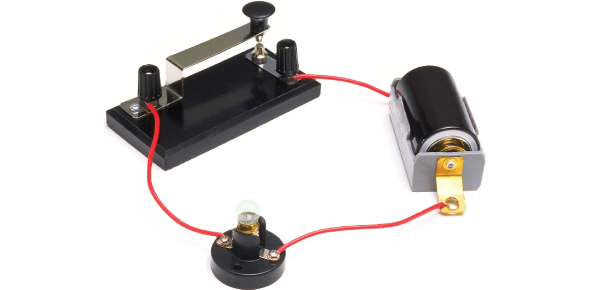# Electric Circuit Logic Gate Quiz: How Well Do You Know?

10 Questions | Total Attempts: 62SettingsDo you know everything there is to know about the Logic Gate? Can you prove your knowledge in this quiz? A logic gate is an idealized model of computation or physical electronic device implementing a Boolean function, a logical operation performed on one or more binary inputs that produce a single binary output. In other words, Logic gates are the basic building blocks of any digital system. It is an electronic circuit having one or more than one input and only one output. Check out the online quiz to see how much you know and learn more with every question you attempt.

• 1.
Is [your statement here] true or false?
• A.

( A + Z` )`

• B.

( A + Z )`

• 2.
What's the expression of this diagram?
• A.

( (A+B) + (C*D) )`

• B.

( (A+B) + (C+D) )`

• C.

( (A*B) + (C*D) )`

• D.

( (A*B) + (C+D) )`

• E.

( (A+B) * (C*D) )`

• 3.
What is the expression of this diagram?
• A.

A` * Z

• B.

A` + Z

• C.

A * Z

• D.

A + Z

• 4.
What is the expression of this diagram?
• A.

(A * Y)` * Z

• B.

(A` * Y)` * Z`

• C.

(A * Y)` + Z

• D.

(A + Y)` * Z

• 5.
What is the expression of this diagram?
• A.

(A` + Y  + Z)`

• B.

(A` + Y  * Z)`

• C.

(A` * Y  * Z)`

• 6.
What is the expression of this diagram?
• A.

A` + Y + Z

• B.

A` + Y + Z`

• C.

A` * Y * Z

• 7.
What is the expression of this diagram?
• A.

( (A * Y)` + Z )`

• B.

( (A * Y)` + Z `)

• C.

( (A + Y)` *Z )`

• 8.
What is the expression of this diagram?
• A.

(A + Y)* D

• B.

(A + Y)* D`

• C.

A * Y* D

• 9.
What is the expression of this diagram?
• A.

(A + B) *C * D

• B.

(A + B) * (C * D)

• 10.
What is the expression shown in the diagram?
• A.

( ( A + B` ) + (C * D) )`

• B.

( ( A + B` ) * (C * D) )`

• C.

( ( A * B` ) * (C + D) )`

Related TopicsBack to top